# Quiz 22: Price Takers and the Competitive Process

Profit is the excess of total revenue over total cost. Total cost is the money value of factors employed in production. There are four factors used in production. They are- 1. Land: It is the gift of the nature that is scarce and used in production. It includes agricultural crops, minerals, animals etc. 2. Labor: it is the manual and mental exhaustion of mankind needed in the production activities. 3. Capital: It is manmade items like machine used in production activities. 4. Organization: It is the special skill of organizing production activities and undertaking risks associated with such activities. Since resources are scarce, some payments are needed to factor owners for using the factors in production activities. Rent is paid to land owner, wages to laborers, interest is paid for capital and normal profit for organization. Sum of these four elements is total cost. Revenue is total sale proceeds. It is ascertained by multiplying price with the quantity sold. Therefore profit is written as-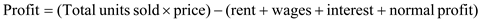Farmer uses factors like fertilizers, machine, labor etc. in the agricultural crop production. If the price of these factors goes up, then price at which produced crop sold in the market will rise. Selling price is inversely related with demand. High price will mean demand will decrease. Fewer crops will be sold. Total revenue will fall and profit will decline. Competitive market can be of two types. They are: 1. Price taker's market and 2. Price searchers market. Price takers have no control over the selling price. Selling price is determined by the market through the interaction of market demand and market supply curve. Market demand curve indicates and inverse relation between selling price and market demand, while market supply curve has a direct relation between selling price and quantity supplied. Price is fixed where market demand is at par with the market supply. A price taker can sell any quantity it likes at the market determined price. Price taker has nothing to do with the determination of price. But a price searchers' market firm has to decide on the quantity to be produced as well as market price. It has a downward sloping demand curve. If it wants to sell more, then it has to reduce price. Price takers are rare. Most of the firms are price searcher. In the problem, farmers are facing a highly competitive industry with low barriers to entry. So they have a price searchers market with a very high degree of competition. Here farmers will be very much sensitive to cost. If price of the fertilizer falls by 10 percent, selling price will also fall. So market demand will go up. Farmers will be able to sell more units to make a reasonable profit. Thus reduction in fertilizer price will be profitable for them.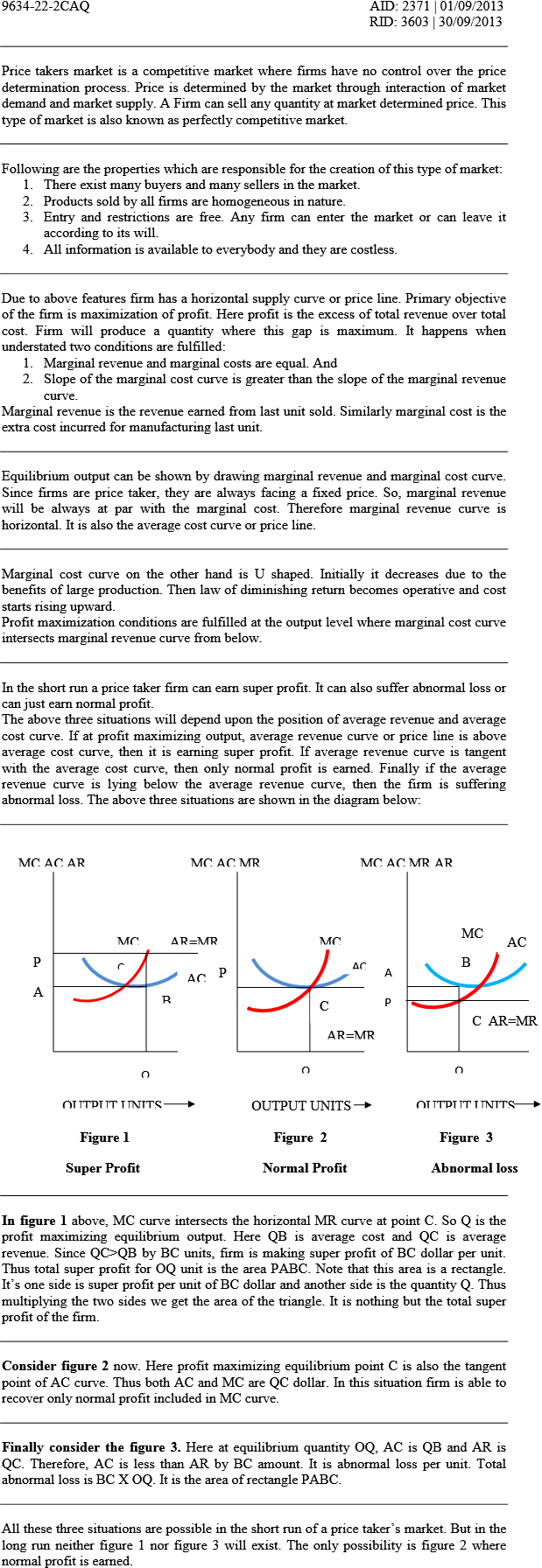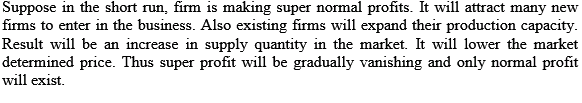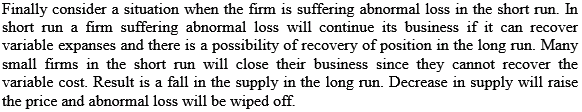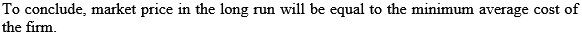In a price taker market it is not guaranteed that the firm will be able to earn at least normal profit even if it can minimize the cost of production. A Price taker market has the understated features: 1. Many buyers and many sellers 2. Homogeneous product manufactured by all. 3. Free entry and free exit. 4. All information is available to every one. 5. Information is costless. Due to the above features firm has no control over the selling price. It is fixed by the market through interaction of market demand and market supply. Market demand is inversely related with price while market supply has a positive relation. Equilibrium price is fixed where the two curves intersect. Market demand and market supply are same at this equilibrium price. Firms in this market operate to maximize their profit. Profit is the excess of total revenue over total cost. A firm will produce a quantity which will enable it to maximize profit. This quantity is known as equilibrium quantity. In determining equilibrium quantity, firm has to sell its product at the market determined price. It can sell any quantity at that price. Thus the firm is said to be a price taker. Profit is maximized when the following two conditions are satisfied- 1. Marginal cost and marginal revenue are equal and 2. Change in marginal cost is greater than the change in marginal revenue. Here marginal cost is the extra cost incurred for manufacturing the last unit. Marginal revenue, on the other hand is the extra money value received by selling the last unit. Equilibrium output determination can be shown by drawing two diagrams. They are marginal cost curve and marginal revenue curve. Marginal curve is graphical representation of marginal cost at different quantity of output. It is U shaped. Thus initially marginal cost will decrease. Then due to imperfect substitutability of factors of production, law of diminishing return will operate and marginal cost will rise. Marginal revenue curve will be linear since price is constant. At profit maximizing output, marginal cost curve will intersect marginal revenue curve from below. Intersection of two curves will mean marginal cost and marginal revenue are same. Intersection of marginal cost curve from below will mean this curve is rising. So its slope is greater than the slope of marginal revenue. Here slope of marginal revenue is positive while the slope of horizontal marginal revenue curve is zero. Now attainment of profit maximizing output level does not mean that firm is at least earning normal profit. It may earn super profit or may even suffer abnormal loss. Ascertainment of abnormal/ normal profit or loss is done by comparing average cost and average revenue. Average will mean per unit figure. It is calculated by the following formulas-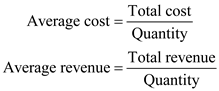Since price is fixed, average revenue will not change. It is also known as price line. If average revenue is greater than average cost firm is making super profit. Here super profit is the excess of profit over normal return. If average revenue is at par with the average cost, just normal profit is earned. It is due to the reason that cost includes normal profit. If average revenue is less than average cost, then firm is not in a position to recover normal profit and thus it is suffering abnormal loss. All these situations may happen in the short run. They are explained in the diagram below: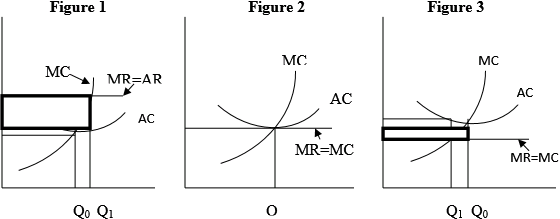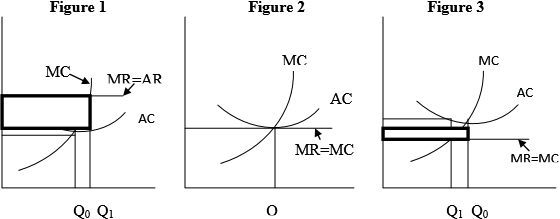In Figure 1, If firm manufacture the quantity which will minimize average cost, then it has to produce OQ ₀ units. It is not maximizing profit. In order to maximize it, firm has to manufacture OQ ₁ units, which is greater than cost minimizing output by Q ₀ Q ₁ units. However even at OQ ₀ units firm is making economic profit indicated by the dark black rectangular area. In Figure 2 , MR=AR curve is tangent at the minimum point of the average cost curve. Also MC curve is passing through this point. Thus both profit maximization output and cost minimization output are equal. It is the situation where firm by manufacturing cost minimizing output is making normal profit. Finally consider the diagram 3. Here AR=MR curve is below average cost curve. Thus whatever units firm will manufacture it cannot even earn normal profit. As the AR is less than AC, firm is always incurring per unit abnormal loss. At cost minimizing output OQ ₀ , it is suffering loss indicated by the thick black rectangle. It will decrease a little if profit maximizing equilibrium output OQ ₁ is produced. Thus in the short run there is no guarantee that a firm in price taker's market will at least earn normal profit by manufacturing cost minimizing output. It can also suffer abnormal loss as shown in the figure 3. However it will not happen in the long run. In long run neither figure 1 not figure 3 will exist. If in the short run firm is making super profit, then new firms will be attracted in the industry. Also existing firm will produce more. Thus total supply in the industry will go up. Market determined price will fall and super profit will be wiped off. Suppose in the short run, firm is suffering abnormal loss. It will mean many small firms will not be able to recover variable cost. They will stop production even when there is possibility of recovery of the situation. Thus industry supply will fall in the long run. Price in effect, will go up. Abnormal loss will abolish. Firm will make only normal profit. So it can be concluded that by producing cost minimizing output, firm cannot guarantee that it will be able to earn at least normal profit. It may even suffer abnormal loss. But in the long run by manufacturing cost minimizing output it can only earn normal profit.